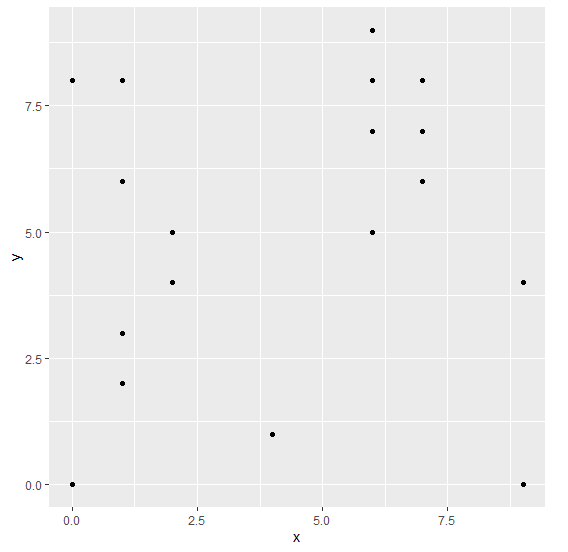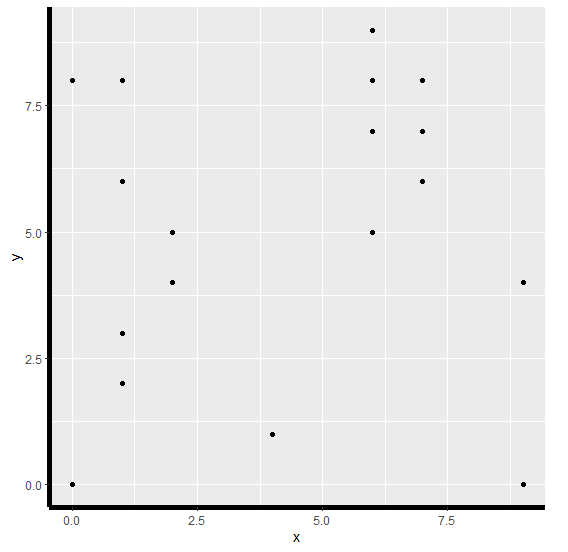# How to increase the width of axes using ggplot2 in R?

To increase the width of axes (both X-axis and Y-axis at the same time) using ggplot2 in R, we can use theme function with axis.line argument where we can set element_line argument to a larger value.

Check out the Example given below to understand how it can be done.

## Example

Following snippet creates a sample data frame −

x<-sample(0:9,20,replace=TRUE)
y<-sample(0:9,20,replace=TRUE)
df<-data.frame(x,y)
df

The following dataframe is created

   x y
1 6 9
2 7 8
3 1 3
4 2 4
5 1 2
6 2 5
7 2 4
8 1 6
9 4 1
10 7 6
11 0 8
12 9 0
13 9 4
14 1 8
15 6 5
16 7 7
17 0 0
18 6 7
19 1 6
20 6 8

To load ggplot2 package and create scatterplot between x and y on the above created data frame, add the following code to the above snippet −

x<-sample(0:9,20,replace=TRUE)
y<-sample(0:9,20,replace=TRUE)
df<-data.frame(x,y)
library(ggplot2)
ggplot(df,aes(x,y))+geom_point()

## Output

If you execute all the above given snippets as a single program, it generates the following Output −To create scatterplot between x and y with increased width of both the axes on the above created data frame, add the following code to the above snippet −

x<-sample(0:9,20,replace=TRUE)
y<-sample(0:9,20,replace=TRUE)
df<-data.frame(x,y)
library(ggplot2)
ggplot(df,aes(x,y))+geom_point()+theme(axis.line=element_line(size=2))

## Output

If you execute all the above given snippets as a single program, it generates the following Output −